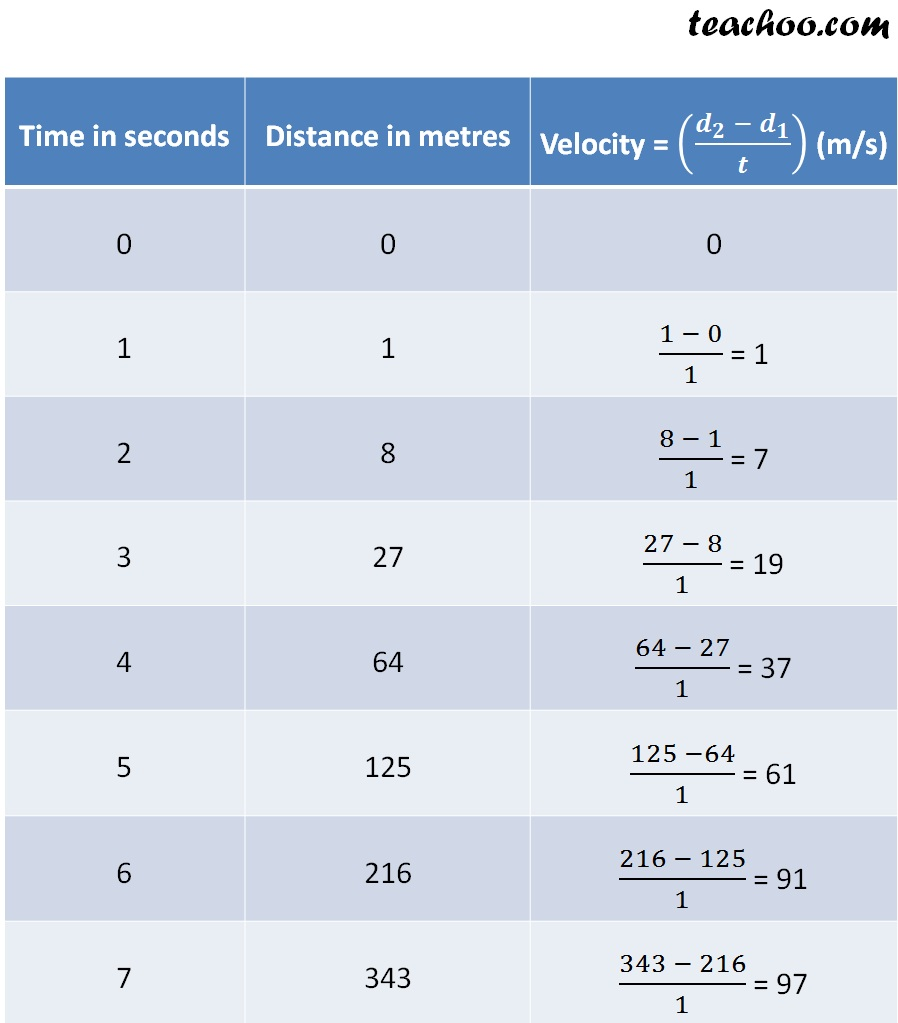Class 9
Chapter 9 Class 9 - Force and Laws Of Motion

## The following is the distance-time table of an object in motion:

 Time in seconds Distance in metres 0 0 1 1 2 8 3 27 4 64 5 125 6 216 7 343

(a) What conclusion can you draw about the acceleration?
Is it constant, increasing, decreasing, or zero?
(b) What do you infer about the forces acting on the object?

(a)

Finding VelocityFrom the above table,

we can see that the velocity changes in unequal amount in equal intervals of time.

∴ The acceleration is non-uniform

Since the velocity is increasing with time,

We can say that acceleration is increasing

So, the acceleration is increasing non-uniformly

(b)

We know that

Force = Mass × Acceleration

As the acceleration is increasing,

the forces acting on the object also increases.

Learn in your speed, with individual attention - Teachoo Maths 1-on-1 Class